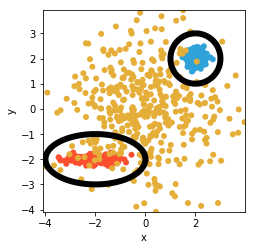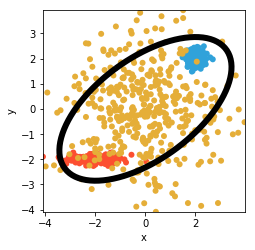# Ellipse¶

Title
Ellipse Element
Dependencies
Matplotlib
Backends
Matplotlib
Bokeh
In :
import numpy as np
import holoviews as hv
from holoviews import opts

hv.extension('matplotlib')An Ellipse is an annotation that takes a center x-position, a center y-position, a size:

In :
opts.defaults(opts.Ellipse(linewidth=6))

# Generate some data
c1 = np.random.normal(loc=2, scale=0.2, size=(200,200))
c2x = np.random.normal(loc=-2, scale=0.6, size=200)
c2y = np.random.normal(loc=-2, scale=0.1, size=200)
c3 = np.random.normal(loc=0, scale=1.5, size=(400,400))
# Create an overlay of points and ellipses
clusters = hv.Points(c1) * hv.Points((c2x, c2y)) * hv.Points(c3)
clusters * hv.Ellipse(2,2, 2) * hv.Ellipse(-2,-2, (4,2))

Out:By default, the size is just a diameter, resulting in a circle such as the blue circle above. Alternatively, the size can be given as the tuple (width, height) as shown for the red ellipse above. If you only supply a diameter, you can still specify an ellipse by specifying an optional aspect value. In addition, you can also set the orientation (in radians, rotating anticlockwise):

In :
clusters = hv.Points(c1) * hv.Points((c2x, c2y)) * hv.Points(c3)
clusters *  hv.Ellipse(0,0, 4, orientation=np.pi/5, aspect=2)

Out:For full documentation and the available style and plot options, use hv.help(hv.Ellipse).